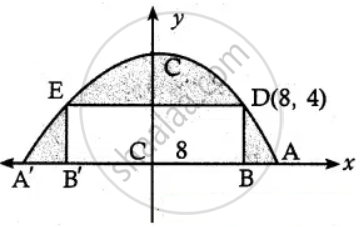Tamil Nadu Board of Secondary EducationHSC Arts Class 12th

# A tunnel through a mountain for a four-lane highway is to have a elliptical opening. The total width of the highway (not the opening) is to be 16 m, and the height at the edge of the road must - Mathematics

Sum

A tunnel through a mountain for a four-lane highway is to have a elliptical opening. The total width of the highway (not the opening) is to be 16 m, and the height at the edge of the road must be sufficient for a truck 4 m high to clear if the highest point of the opening is to be 5 m approximately. How wide must the opening be?

#### SolutionLet the equation of the ellipse be

x^2/"a"^2 + y^2/"b"^2 = 1

Length of semi minor axis b = 5

i.e., x^2/"a"^2 + y^2/5^2 = 1

Let BB’ be the road width and AA’ be the endpoints of the opening of the tunnel.

Let CB = 8, BD = 4

∴ D is (8, 4) lies on the ellipse

8^2/"a"^2 + 4^2/5^2 = 1

⇒ a2 = 25/9 xx 64

⇒ a = 40/3

The width AA’ = 2a

= 80/3

= 26.66 m

The required width is 26.66 m

Concept: Real Life Applications of Conics
Is there an error in this question or solution?

#### APPEARS IN

Tamil Nadu Board Samacheer Kalvi Class 12th Mathematics Volume 1 and 2 Answers Guide
Chapter 5 Two Dimensional Analytical Geometry-II
Exercise 5.5 | Q 2 | Page 214
Share